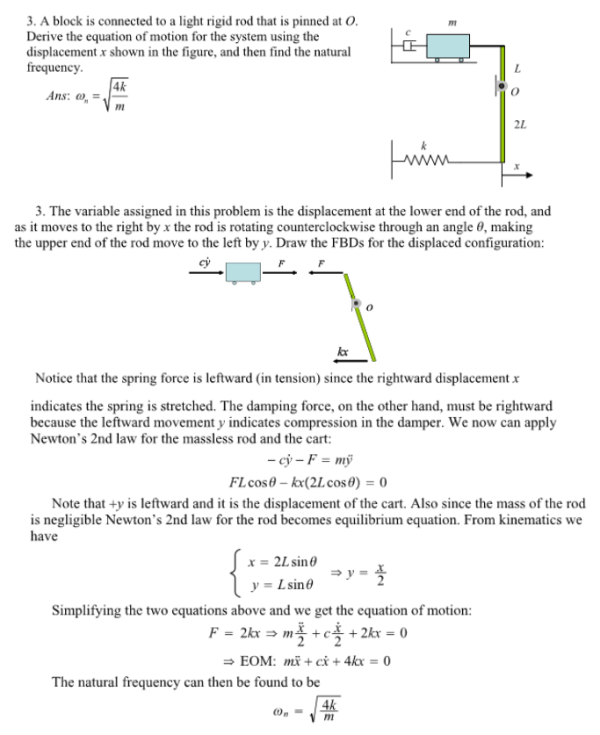A block is connected to a light rigid rod that is pinned at O. Derive the equation of motion for the system using the displacement x shown in the figure, and then find the natural frequency.A block is connected to a light rigid rod that is pinned at O. Derive the equation of motion for the system using the displacement x shown in the figure, and then find the natural frequency.

System Dynamics Page 2 dynamics dynamics dynamics dynamics dynamics dynamics dynamics System dynamics Page 3